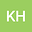loading page

Computing Generalized Linear Model using Iteratively Weighted Least Squares and Coordinate Descent
••• Khoa Huynh,
• Xia Wang
Khoa Huynh
Department of Mathematical Sciences Division of Statistics and Data Science, University of Cincinnati
Author ProfileXia Wang
Department of Mathematical Sciences Division of Statistics and Data Science, University of Cincinnati

## Abstract

Generalized Linear Model (glm) has been widely used in regression models. Iteratively weighted least squares algorithm (IWLS) and coordinate descent (CD) algorithm are technique that can used to nd maximum likelihood function for generalized linear model. We are interested in comparing the two algorithm to the framework of regression models with binary responses. Link function is an important component in the generalized linear model. We carried out a simulation study to compare glm using IWLS algorithm and CD algorithm performance under dierent link functions. The link functions we investigated include the commonly used logistic (logit), probit and complementary log-log links (cloglog). We also wrote an algorithm glm using iteratively weighted least squares and coordinate descent algorithm. Our results show that under IWLS algorithm perform better than glm under CD algorithm when we increase the number of variable. The current study helps our future research to build an integrated process of variable selection via lasso along with a exible link function.